Lifetime Value (LTV) is one of the most important metrics we follow in SaaS. It tells us the value our customers bring to our business.

We need it to make informed decisions about customer acquisition expenses. For example--we shouldn't spend more than we expect to earn. But how much do we gain? How accurate are our LTV estimates? Do our estimates get close to the real thing?

Typically, LTV is estimated using a "simple" LTV calculation. It's ubiquitous in our industry: a simple but effective metric for estimating LTV. But is it really effective?

In our previous post, we explored pitfalls in using "churn" metrics. We found that "churn" misleads us in some circumstances, such as when there are many short-term customers.

Our most popular metric for LTV is a "churn"-based metric, where churn is in the denominator when calculating it. So what does that say about our LTV calculations? Are they wrong sometimes?

In this post, we're going to test the "simple" LTV metric. When is it accurate? Where does it mislead us? How close does it get us to the actual LTV? Read on to find out.

Before we dive in, let's review what "simple" LTV is. Here is the formula below:

LTV = Average monthly revenue per user/user churn

Notice that it includes "churn" in the denominator. That "churn" is the same metric we tested in our previous post.

If churn misleads us for customer retention, does it also mislead us for lifetime value calculations? Let's investigate! As usual, we'll turn to simulations to answer the question.

Let's run some simulations where we know the actual value for LTV and see how the "simple" method performs at estimating it.

## Scenario 1: LTV in a stagnant business with many short-term subscribers

Let's simulate a simple business situation: a stagnant business, where nothing is changing. Sign-ups stay the same. Dropouts stay the same, and the average lifetime of a subscription does not change. Here's a summary:

• Average sign-ups per month: 30
• Average subscription lifetime: 2 months
• Subscription price: \$20/month
• Many short-term subscribers

The graph below illustrates a simulation where we generate the data ourselves using known parameters (such as sign-up rates, subscription lifetimes). Since we created these features, we know the ground truth. We can evaluate our metrics, such as “simple” LTV, by comparing them against the truth.

Here's a look at the active subscriber population in the simulation we described above: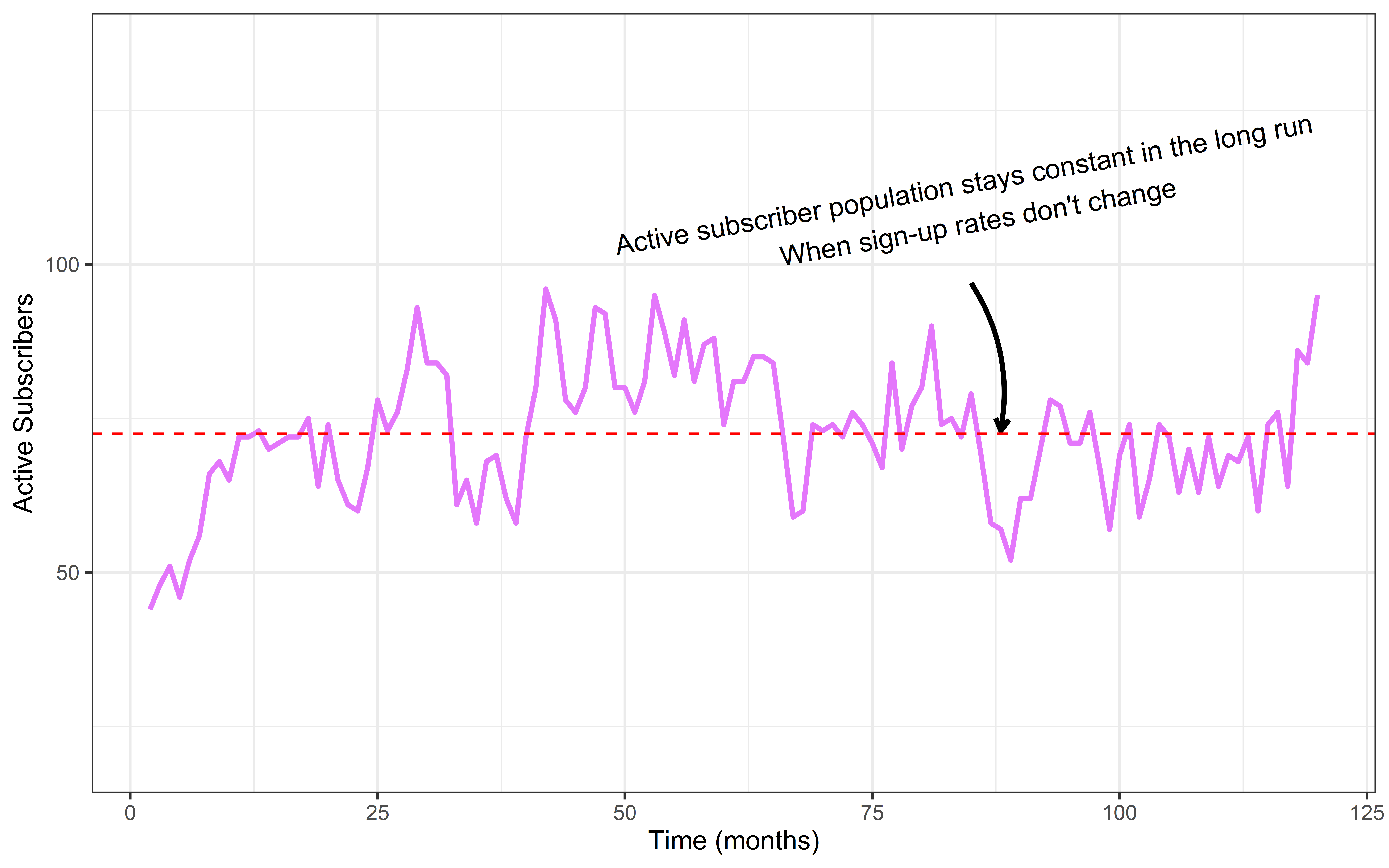Notice how the population of active subscribers grows quickly over the first 20 months, then the population fluctuates above and below the red dotted line for the rest of the time in the simulation. After about 15 months, the business is truly stagnant. The purple active-subscribers line will hug the red dotted line forever, fluctuating at around 72 subscribers.

The stagnant business scenario should be easy for an LTV metric to handle because nothing is changing. There should be plenty of data for the metric to capture the true LTV. But can our "simple" LTV metric approach the true LTV that we know?

Let's calculate LTV each month in the time series above. We will begin with small amounts of data in the early months, but as our dataset grows, the estimate should get closer to the real LTV value. Does it?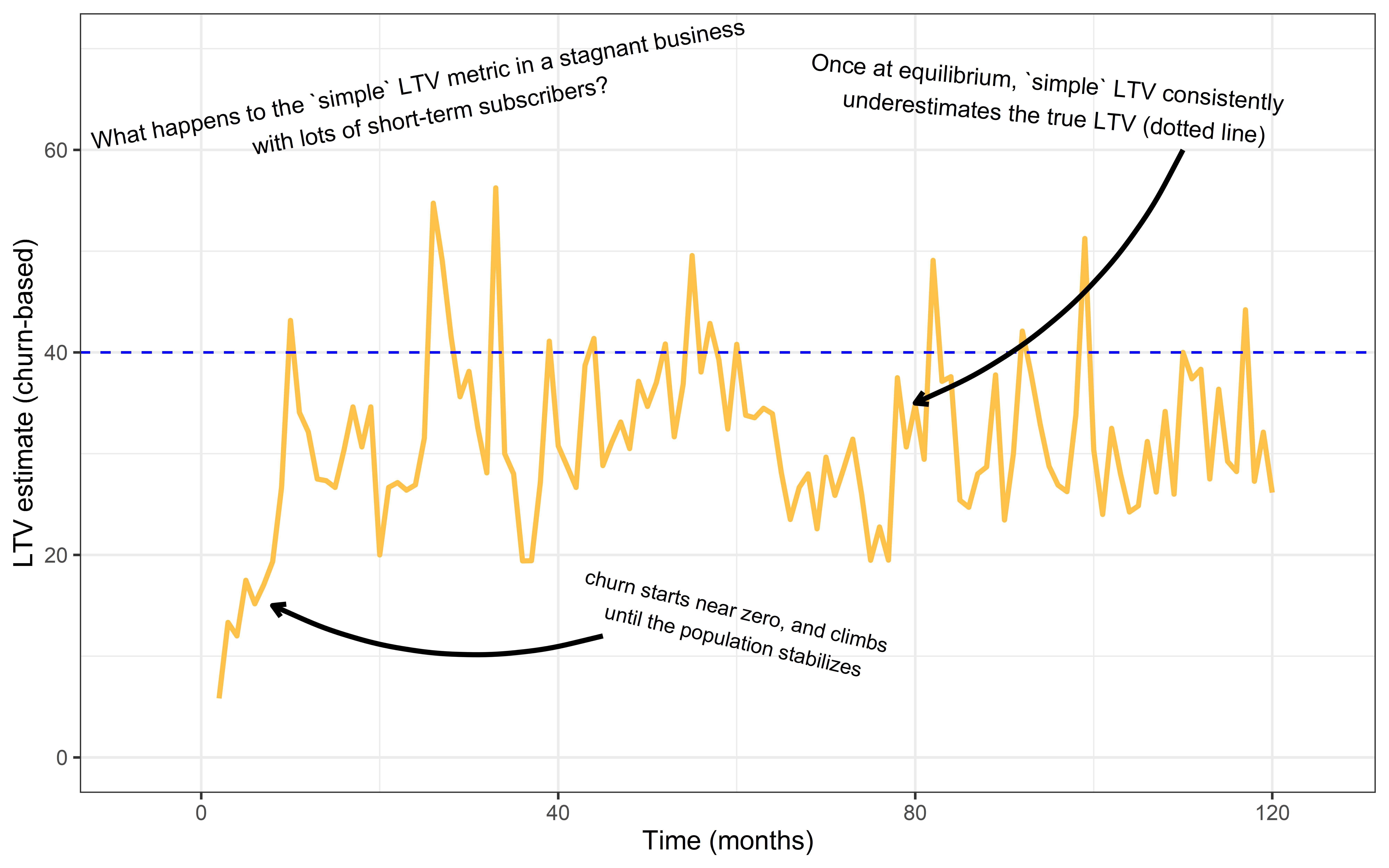The chart above shows the simple LTV estimate calculated every month. The blue line indicates the actual lifetime value for this customer base. Notice that while the business is in its growth phase, our “simple” LTV underestimates the true LTV by a lot.

Just to recap, we know the LTV in this simulated situation as we have chosen it. We then used the simple LTV metric to calculate LTV. Then, we compared the simple LTV metric and the LTV metric that is a parameter of the simulation to see if they were the same or not.

The simple LTV starts near zero. It starts small because the customer population hasn't stabilized yet, and many short-term customers are dropping out relative to the rest of the customers.

The simple LTV is much closer to the real thing when the population stabilizes, but we still tend to underestimate LTV. The average LTV estimate when the population stabilizes is \$32. That's 20% less than the true value, \$40. It's an underestimate, but surprisingly, it isn't terrible!

While not perfect, simple LTV calculations in the above scenario seem usable, being only about 20% off.

But how does the "simple" LTV calculation fare in a more typical Baremetrics user's customer base, where customers subscribe for an average of a few years?

## Scenario 2: LTV in a stagnant business, long-term subscribers

What if we tried the simulation with a different customer base? Would we still get under-estimates? Let's try a new simulation with fewer short-term subscribers. Would our LTV estimate be any better?

The graph below represents a different simulation. The parameters are the same as before, except for a different distribution of subscribers. This population closely resembles an actual Baremetrics customer.

In this simulation, there are fewer short-term subscribers, and the average subscription lifetime is 3.7 years. If the average subscription lasts for 3.7 years, then the actual LTV must be \$904.

This is calculated by doing 3.7 years * 12 months * \$20 per month.

Can "simple" LTV get close to that \$904 number?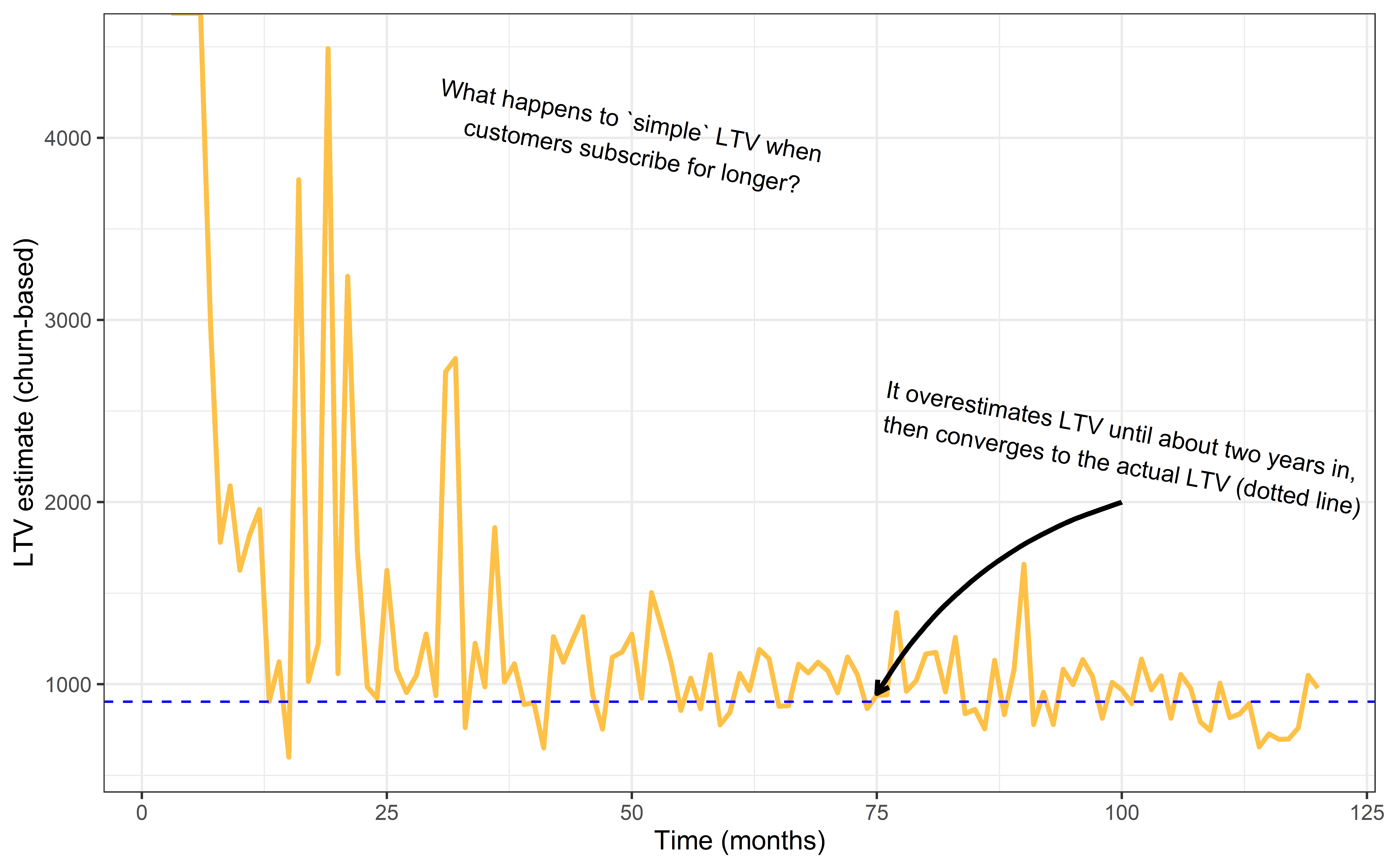In the above chart, the yellow line represents the simple LTV calculation, calculated each month as the simulation progresses. Notice that we get dramatic over-estimates at the beginning. Why is this? It's because we have more longer-term subscribers. In the early months of this process, very few of the customers are dropping out. As a result, our estimate for LTV is much too high because our estimates expect the subscriptions to last forever with such a low drop-out rate.

The customer population stabilizes after 3.7 years, however. After 3.7 years, we get estimates close to the actual LTV (blue line). What's more, it appears as a much more accurate estimate than in our previous scenario. It's off by only a few percentage points!

So as a verdict, "simple" LTV is not so bad in the case where customers stay long-term, and nothing is fundamentally changing in the business! Especially considering the issues, we discussed in our previous post about churn.

So can we find a scenario where "simple" LTV fails?

Maybe "simple" LTV fails when the customer population is rapidly changing, just as churn failed in our previous post.

Perhaps we see the same problem here because our simple LTV estimate wildly overestimates the lifetime value for the first two years.

## Scenario 3: LTV in a growing business

Let's try another simulation to explore this issue further. Let's keep everything the same as the scenario we did above, but with one difference:

Suppose we have a great marketing team that steadily increases the number of sign-ups each month.

Let's suppose that each month, our average sign-up rate goes up by one percent.

After 100 months, we should be getting double the customers on average each day. The lifetime value, however, doesn't change. Does this extreme growth ruin our LTV estimate?

Here is a chart of the active customer base: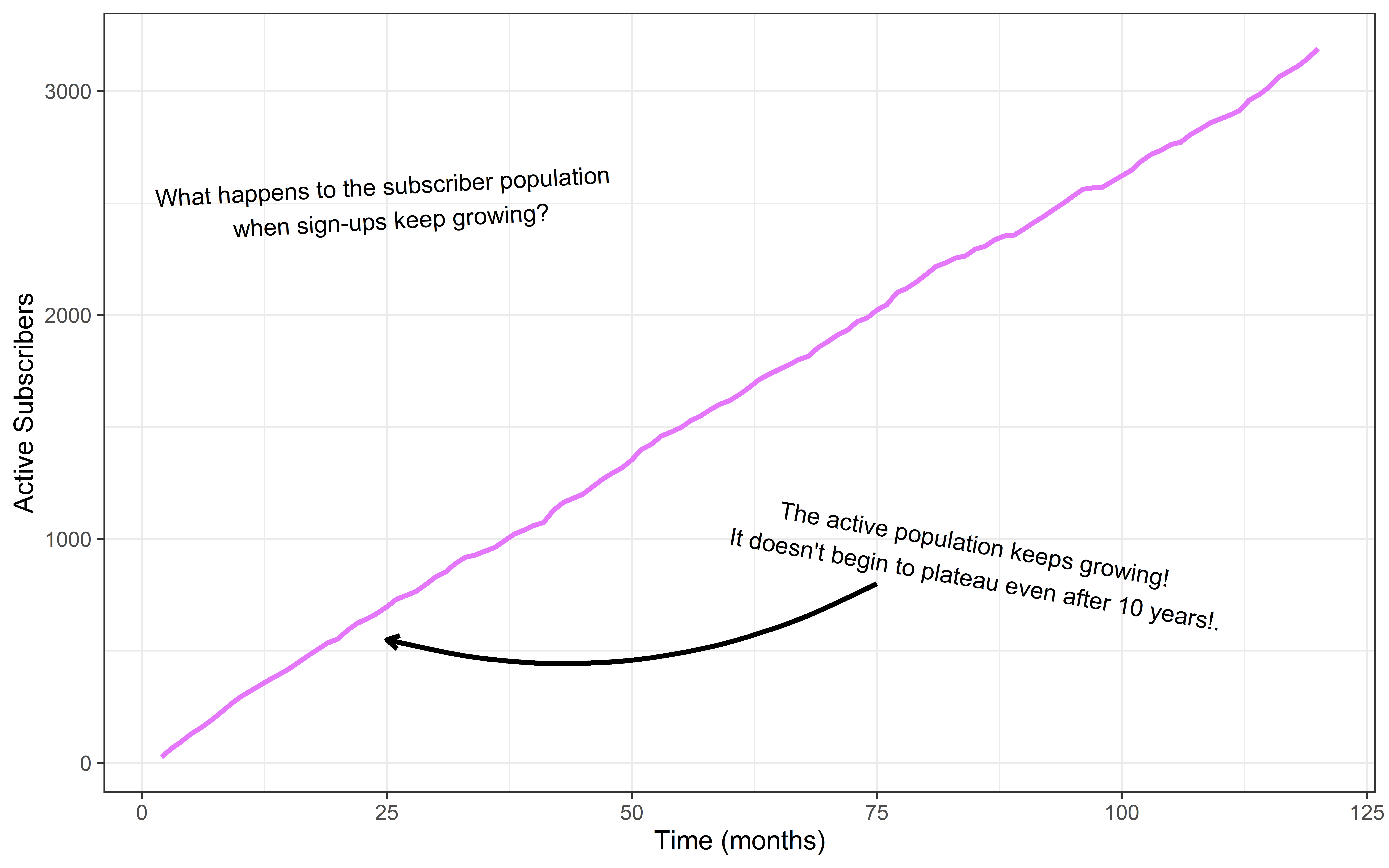As we get increasing sign-ups, our active customer population rockets upward.

Amazing! How does our LTV calculation fare?

See the chart below: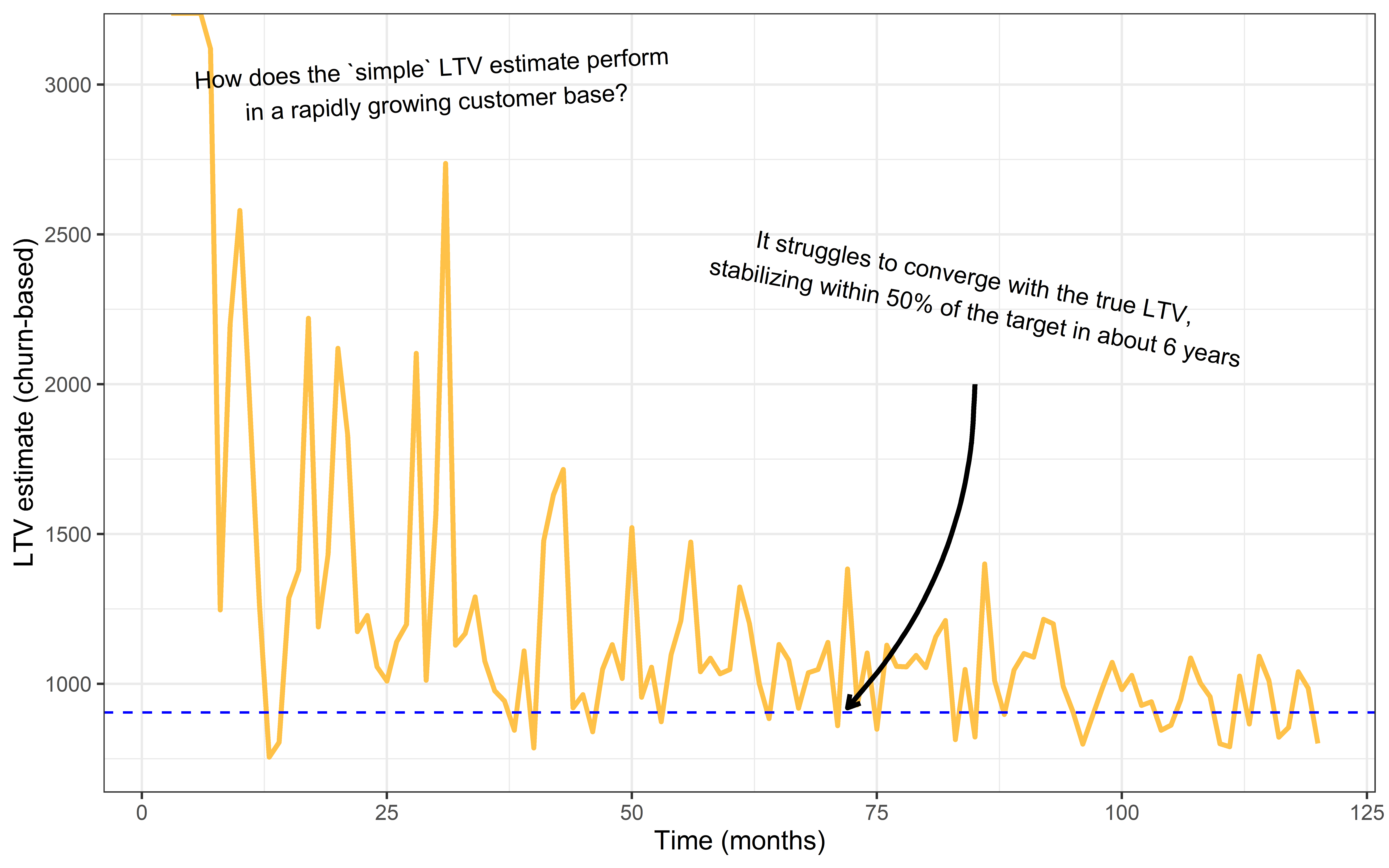In the above chart, we see how "simple" LTV estimates (yellow) take longer to converge with the true value for LTV (dotted line).

The estimates do eventually converge with the true value, but only after about ten years. Even five years into this time series, our LTV estimates are off by as much as 50%.

It appears the "simple" LTV estimate is sensitive to a changing customer population, even though the customer population size should have nothing to do with the lifetime value of the individual.

This sensitivity to population size is because the simple LTV estimate depends on churn, and churn is sensitive to a rapidly changing population size.

## Let's try a different metric for LTV

Can we find a better metric for LTV? One that doesn't get distorted by changing sign-up rates? Instead of the simple LTV estimate, let's build one based on statistics.

How about this: we estimate the average lifetime of a customer using a statistical model. We'll build a statistical model for subscription durations using our data.

We'll be using Weibull regression as our statistical model. We feed data into the model, and it will spit out an estimate for the average subscription lifetime.

Then we multiply the average subscription lifetime by our monthly rate of \$20/month to obtain an LTV estimate. How would such an estimate compare with "simple" LTV?

Let's repeat the previous simulation with growing customer sign-up rates and calculate the LTV both ways to compare.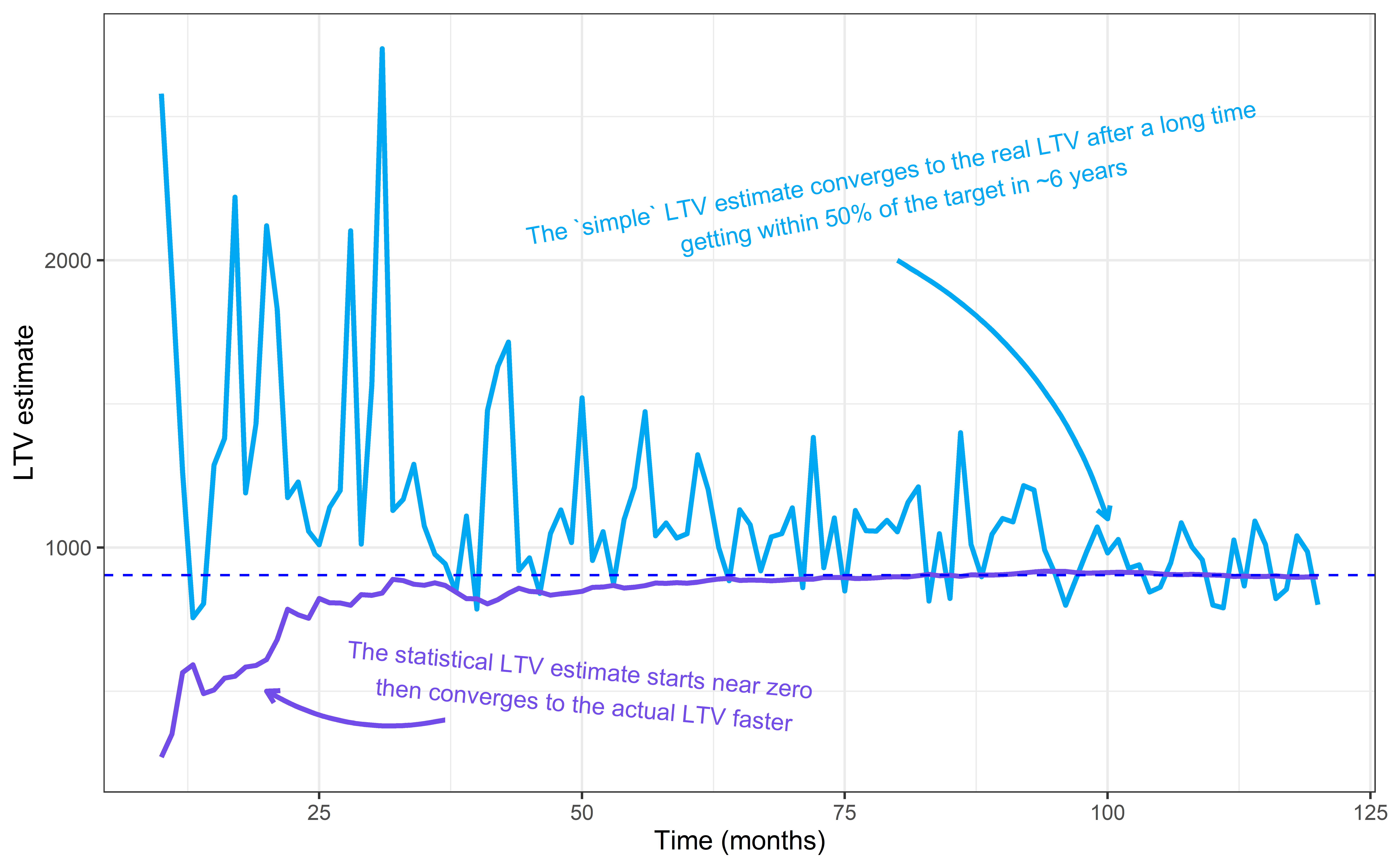In the plot above, we show LTV estimates over time. The "simple" LTV estimate is blue, and the statistical estimate is purple.

Notice how the statistical method converges with the true LTV value (dotted line) long before the "simple" estimate. With the statistical estimate, we get within a few percentage points to the actual LTV with about 18 months of data, long before the customer base reaches a stable population.

In contrast, the churn-based estimate takes ten years to catch up and never reaches the same level of accuracy. The statistical estimate outperforms the simple churn-based one.

## Statistics beats "simple" LTV, but can we do better?

An interesting side-note: Notice how at the beginning of the above chart, the churn-based estimate over-estimates the actual LTV, whereas the statistical method underestimates it. Why? Both of these distortions happen because these estimates are naive. They don't know anything before seeing data, so they begin with absurd estimates: the statistical estimate starts at zero, and "simple" churn starts near infinity.

Can we improve our estimates by giving them a better starting point before they see any data? Yes!

It turns out we can improve our statistical estimate by giving the model some education. Suppose the model starts with a vague idea of what an average subscription lifetime looks like, based on previous experiences or experiences of peers. Let's say we expect a subscription lifetime to last about a year. A wide ball-park guess, but more realistic than zero. Then as the data rolls in, we update our estimates.

As we collect more data, our vague starting point will steadily disappear, giving way to whatever the data tells us. But in the beginning, it will help by starting closer to the truth.

What we are doing is called regularization in statistics and machine learning. It's a technique where we add bias to our model--the good kind of bias. It puts more weight on realistic data points, and it treats extreme observations with skepticism. We get better estimates as a result.

Let's try it out! Below, we've added a new, improved estimate that uses regularization. It's similar to the statistical estimate, but it makes use of something called a Bayesian prior.

A prior is like a vague pre-conception. It nudges our estimates towards what we know to be reasonable subscription lifetimes, so our estimates start closer to the real thing. Let's see how it does: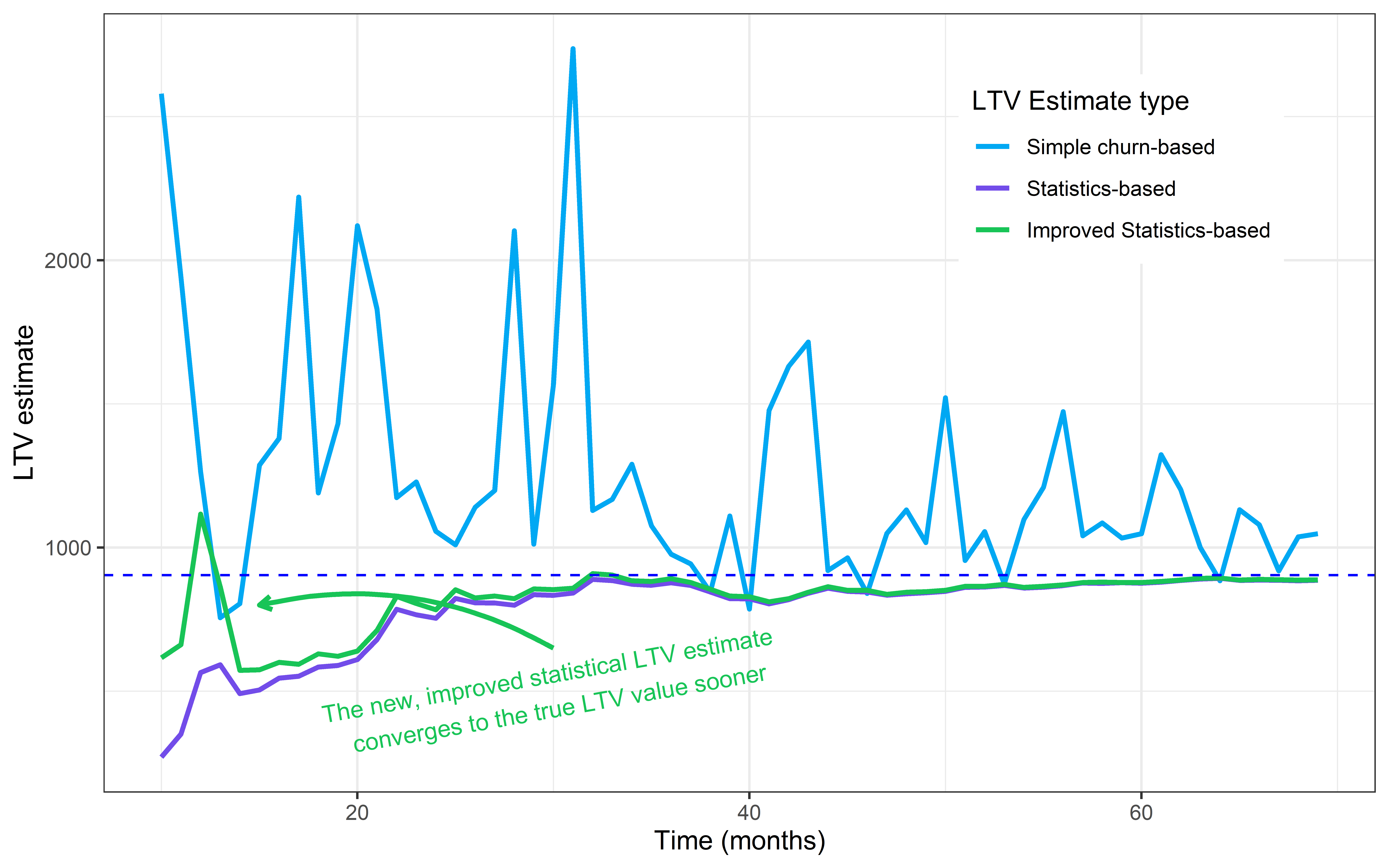In the above chart, we’ve added an improved statistical estimate (green). Notice how the new estimate outperforms the other estimates in the first few months. This improvement does exactly what we intended.

Our improved estimate is closer to the real LTV value in the early months. As the data rolls in, however, the two statistical estimates converge, eventually becoming indistinguishable.

Hopefully, we have shown that it is possible to find better LTV metrics than churn-based ones. Churn-based LTV estimates can be highly inaccurate, especially when there is little data. We can replace it with these better metrics. Further, we can also fine-tune them, so they are better suited for our field in SaaS.

In future posts, we will explore these statistics-based metrics further. We will test their limits and see how they could be helpful to us for business intelligence. Stay tuned!